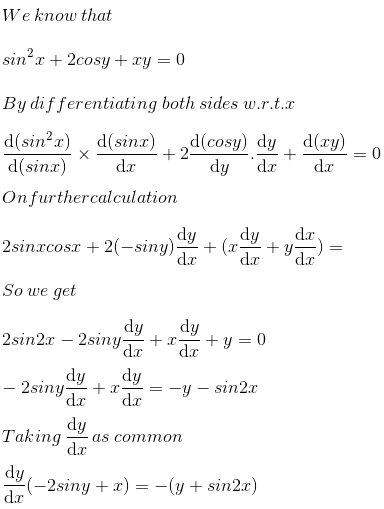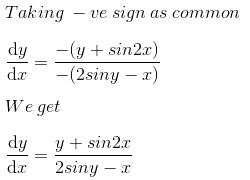# RS Aggarwal Solutions for Class 12 Maths Chapter 10: Differentiation Exercise 10E

Exercise 10E of Chapter 10 explains the concept of differentiation of implicit function relevant to the current CBSE board. Students who aspire to score well in the board exam can solve the exercise wise problems using the solutions which are available for free download. The solutions designed by teachers at BYJU’S give a clear idea about the concepts, which are covered under each exercise. Students can download RS Aggarwal Solutions Class 12 Maths Chapter 10 Differentiation Exercise 10E PDF from the links available below.

## RS Aggarwal Solutions for Class 12 Maths Chapter 10: Differentiation Exercise 10E Download PDF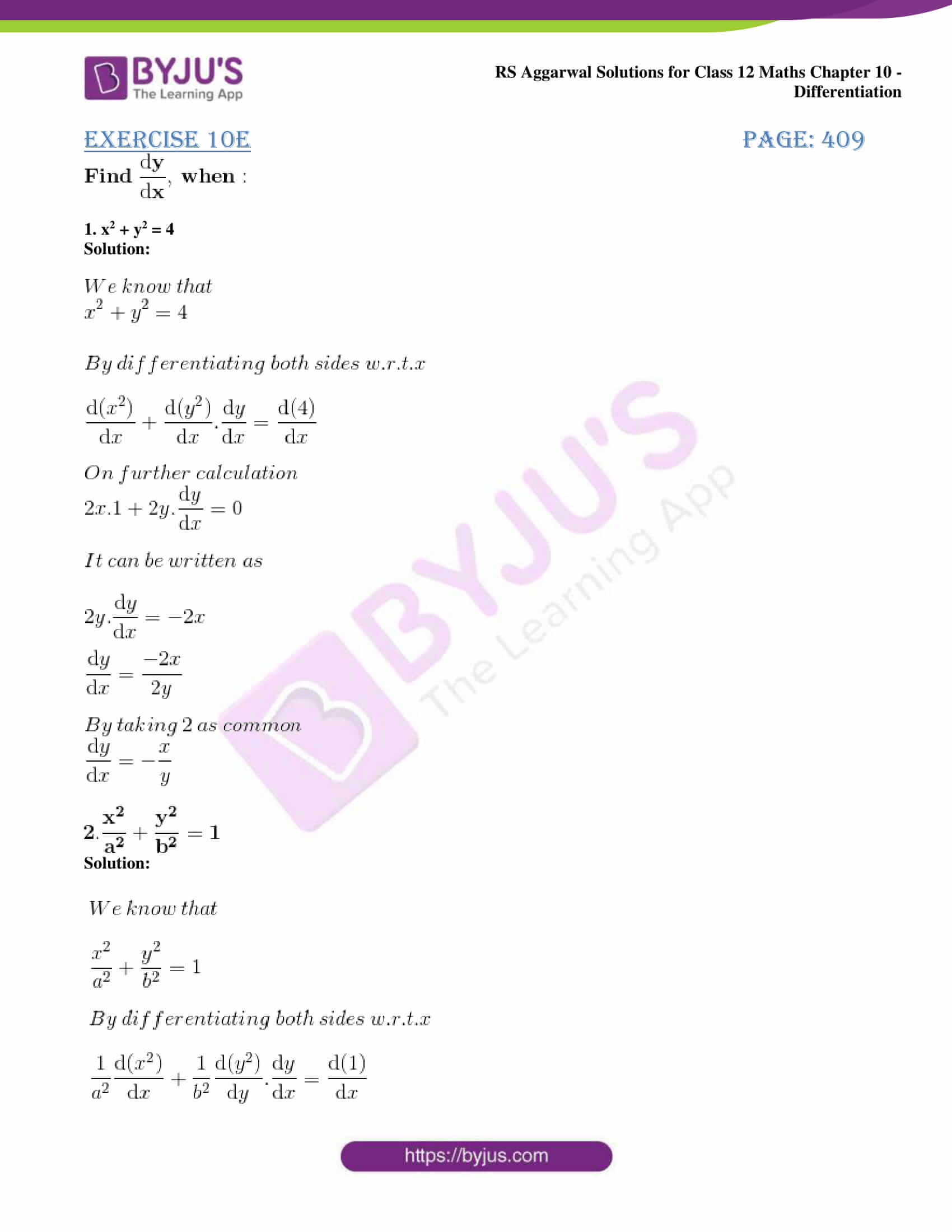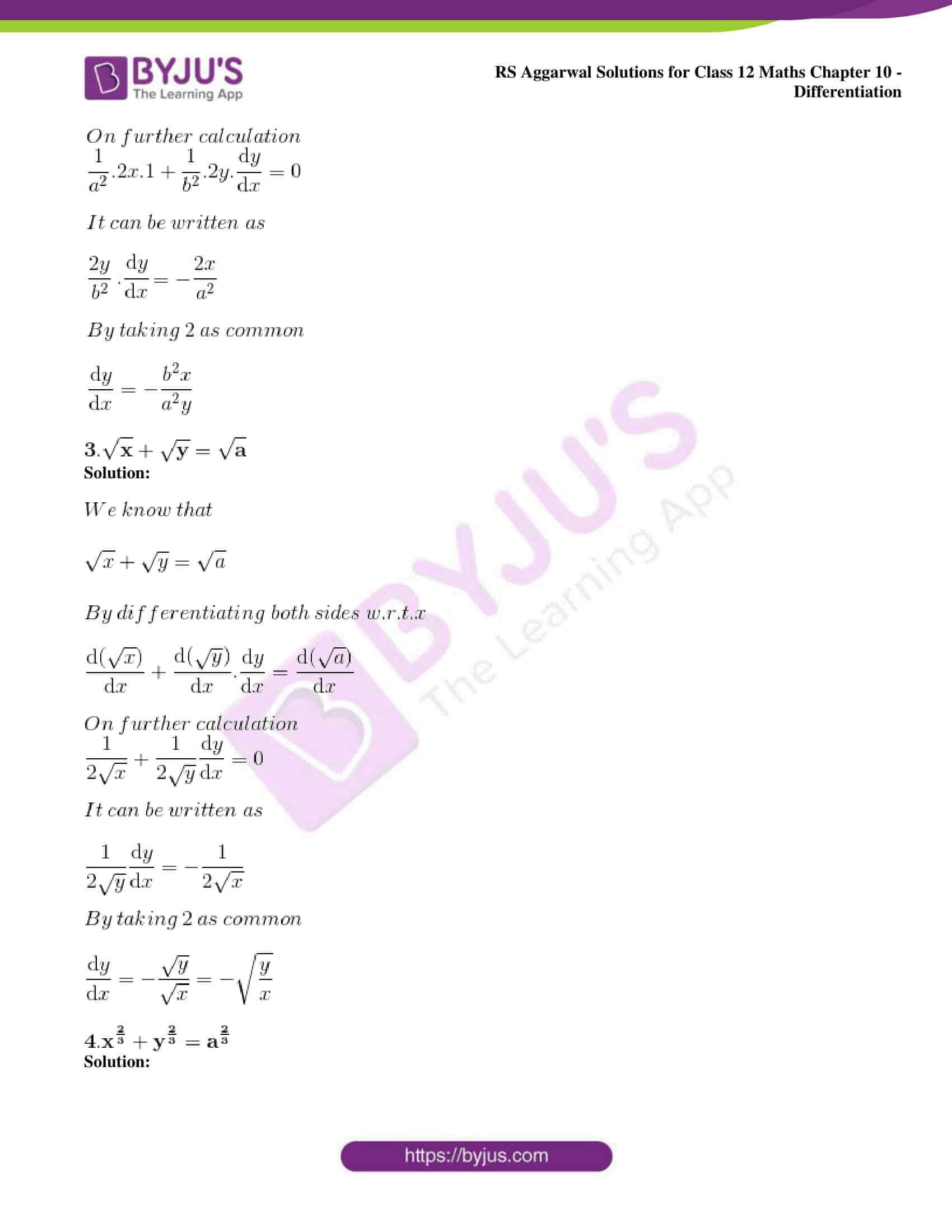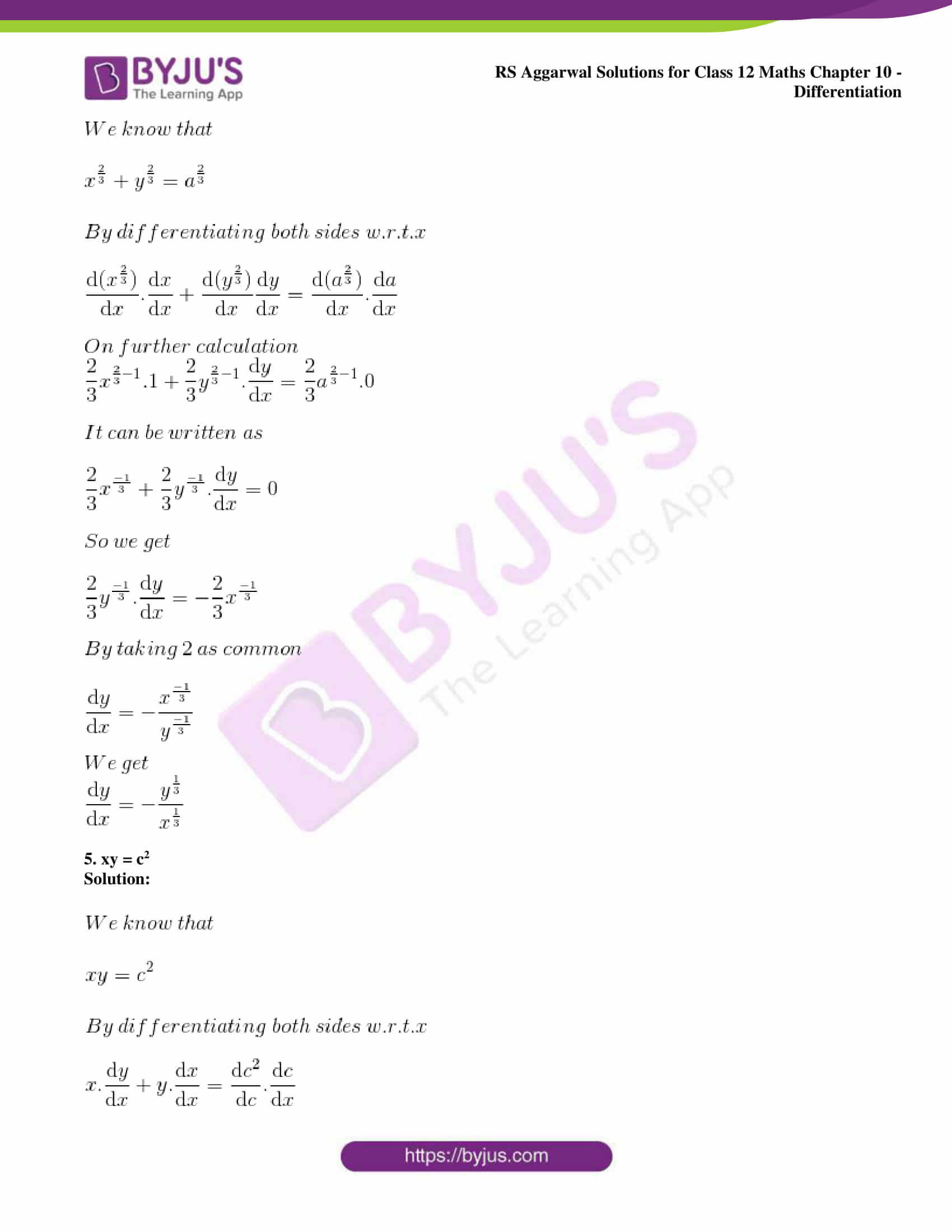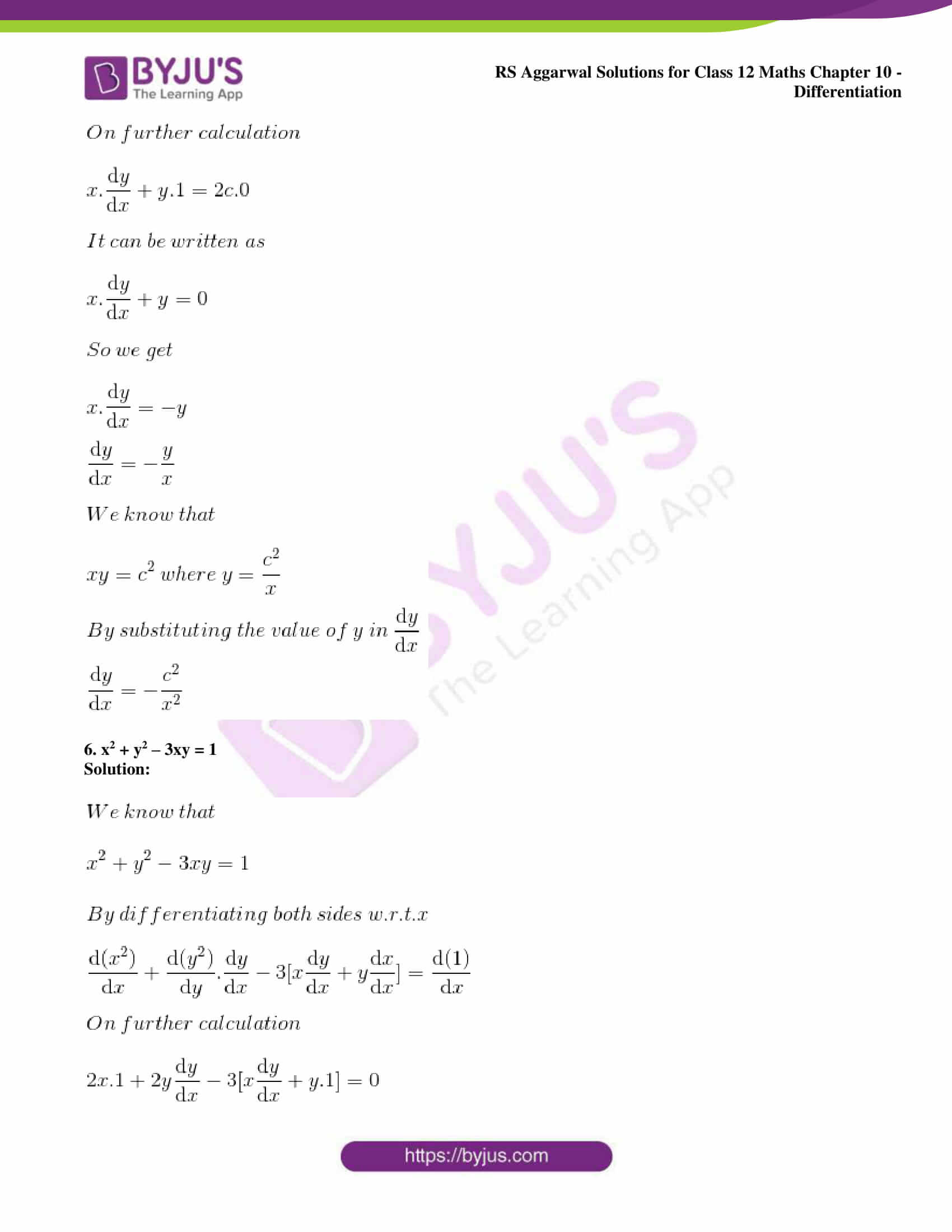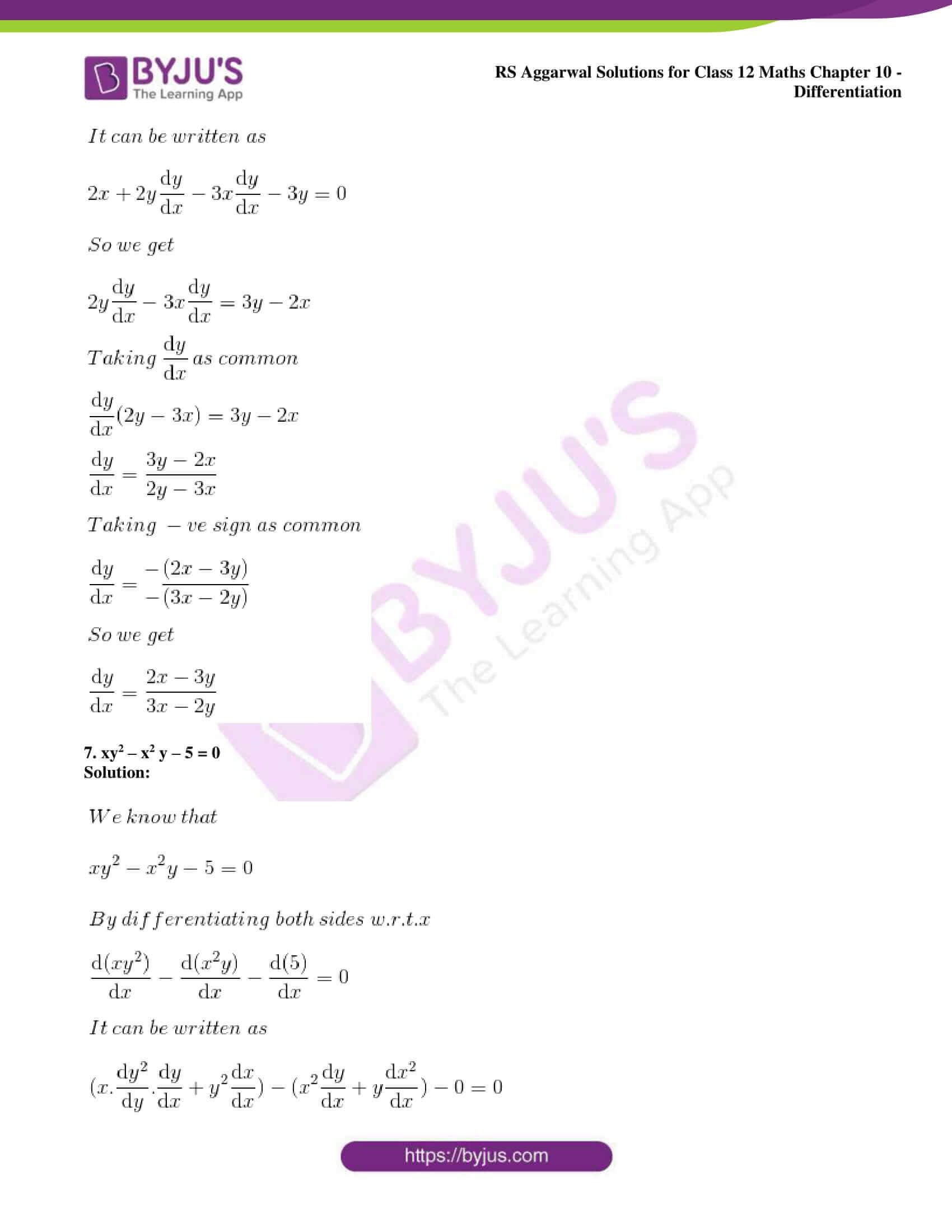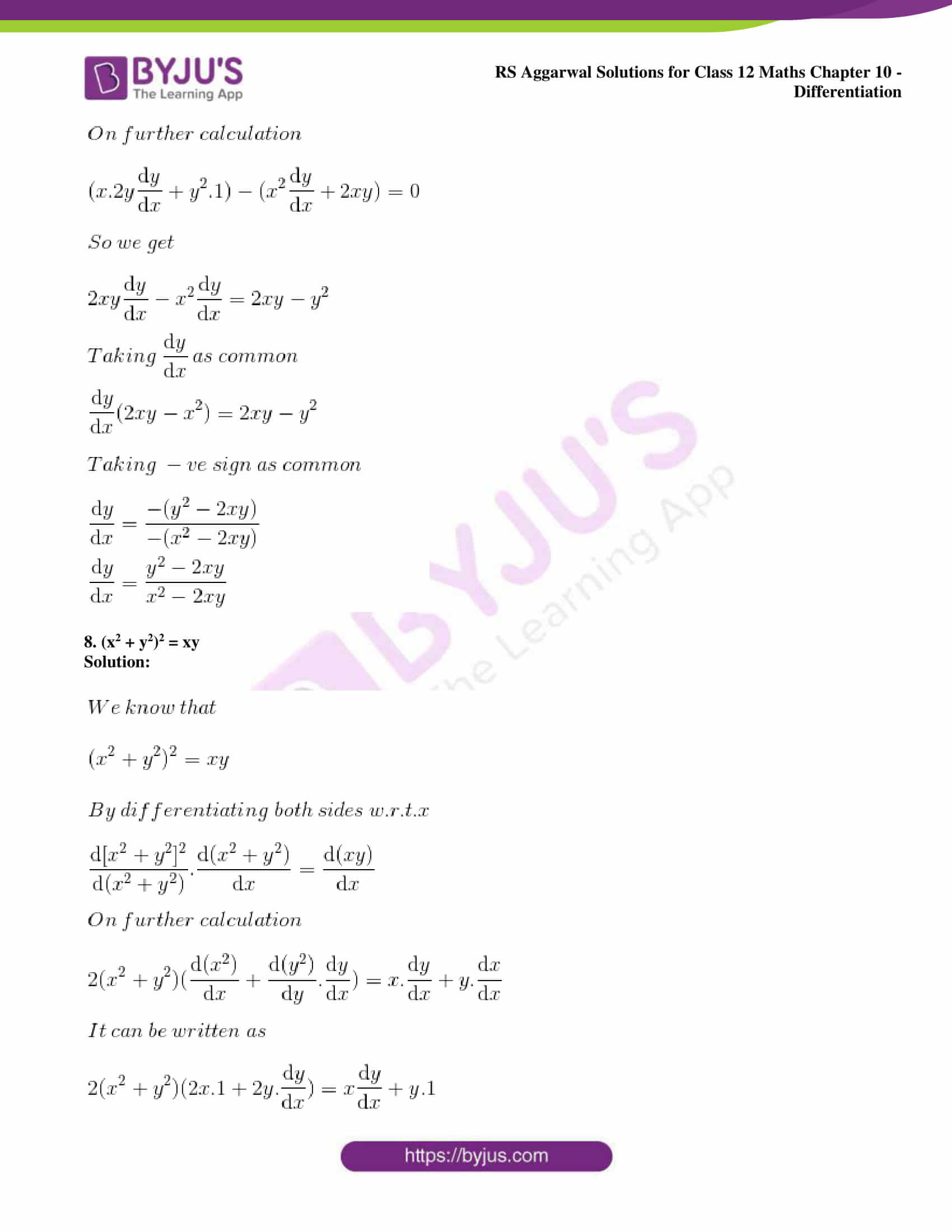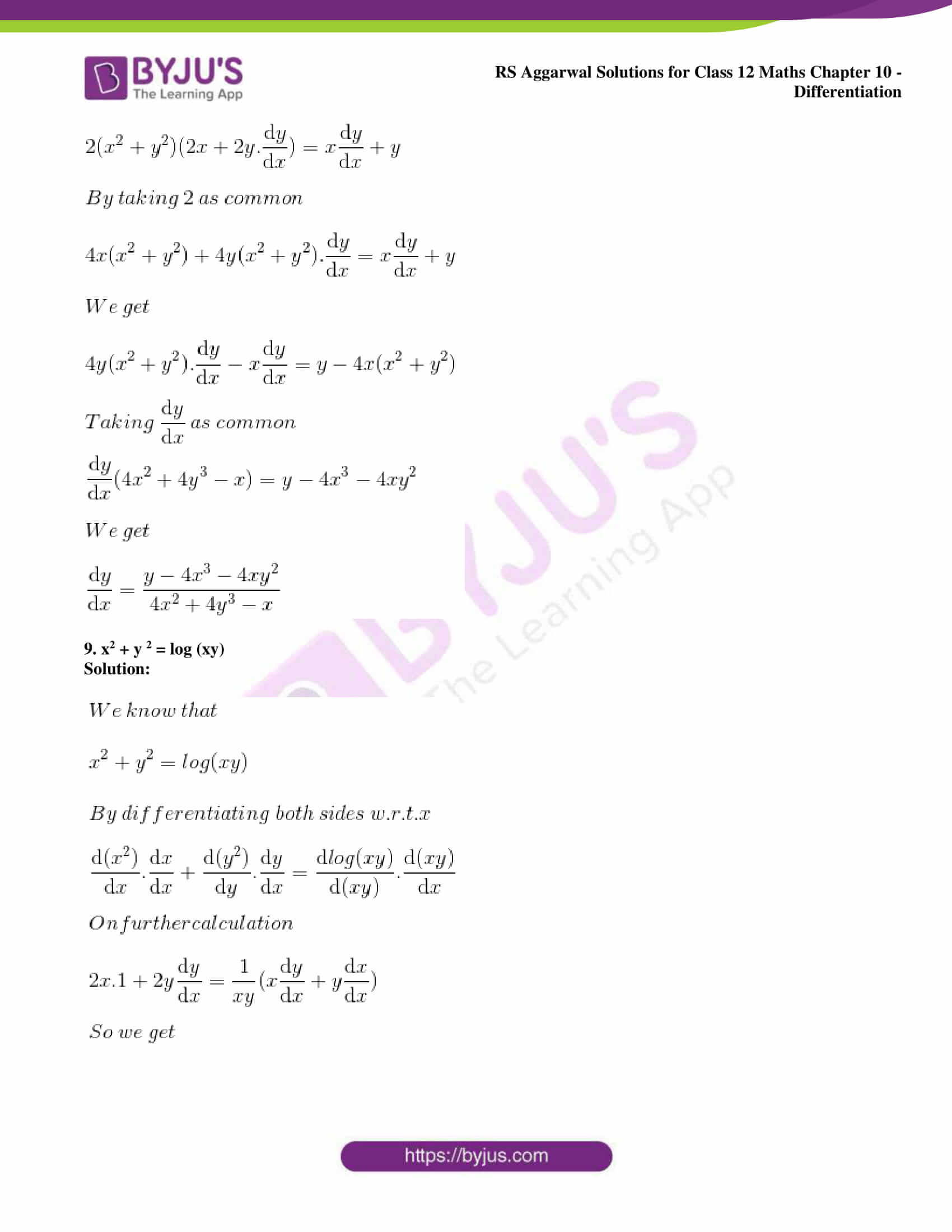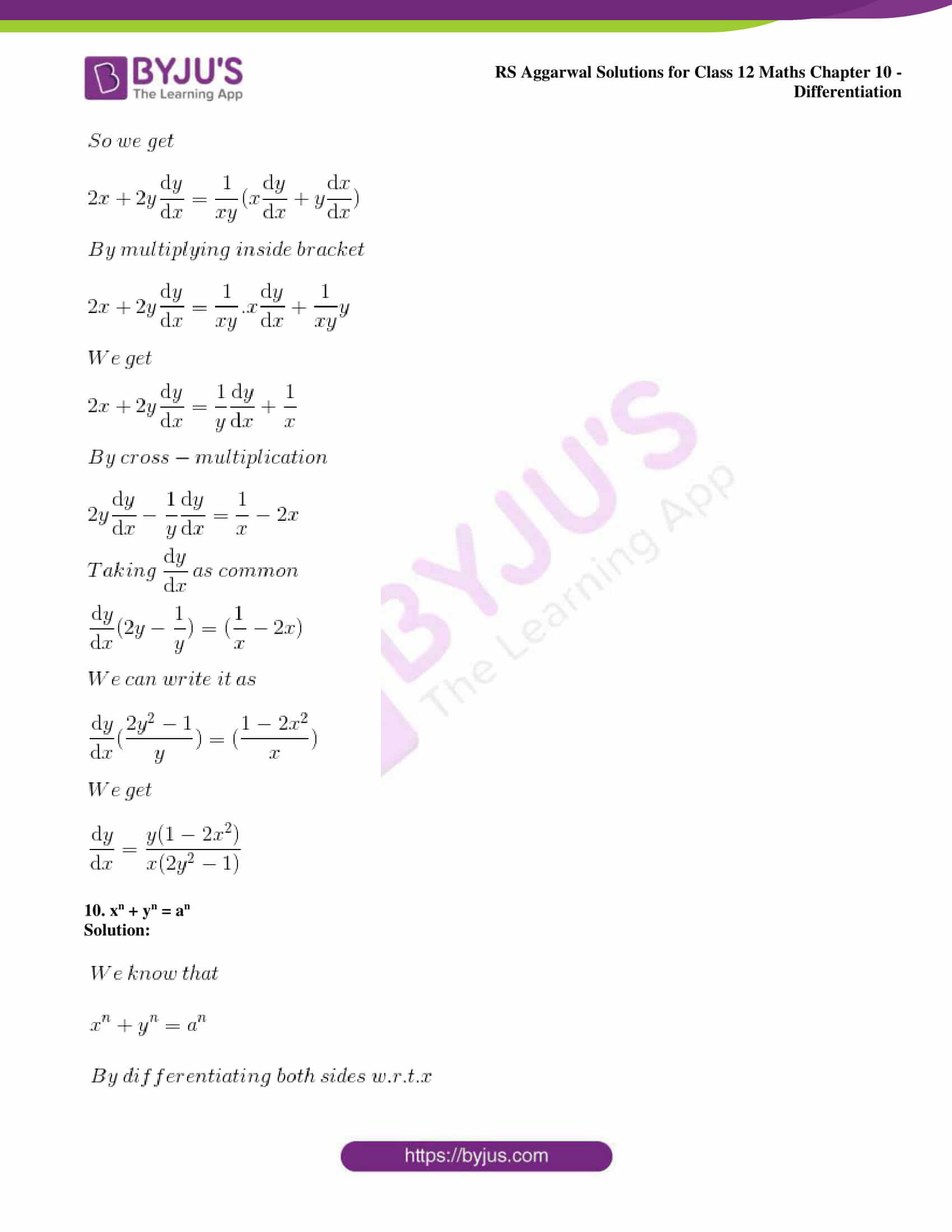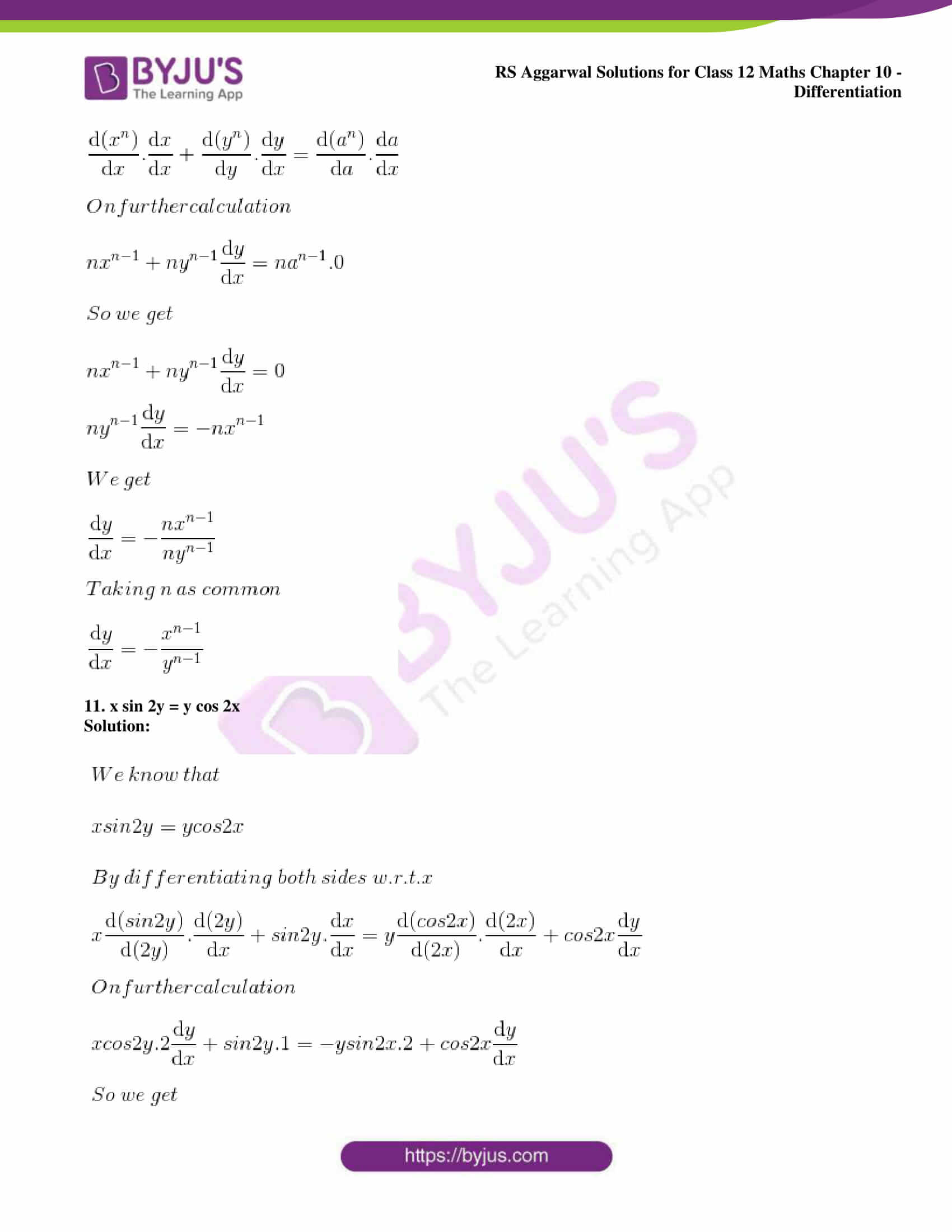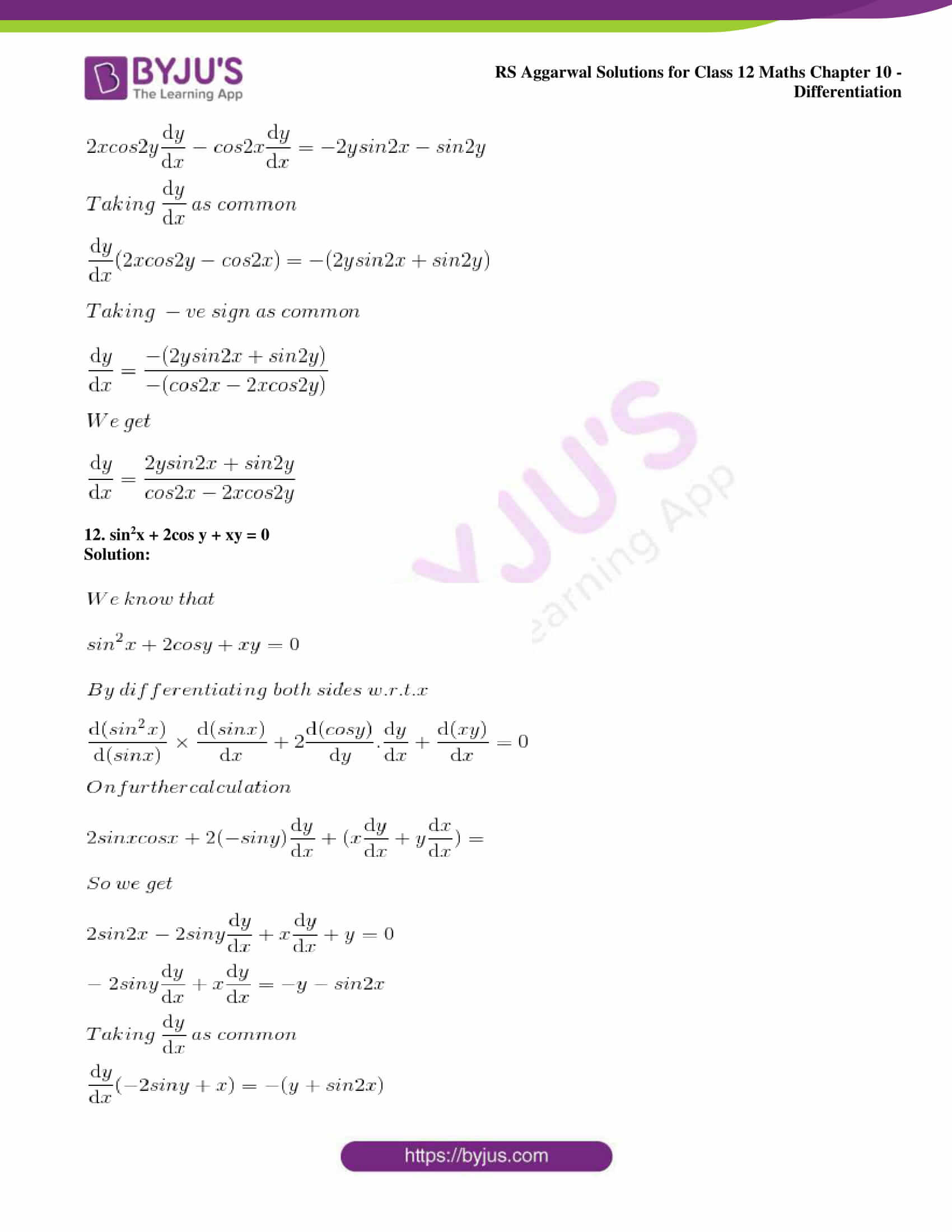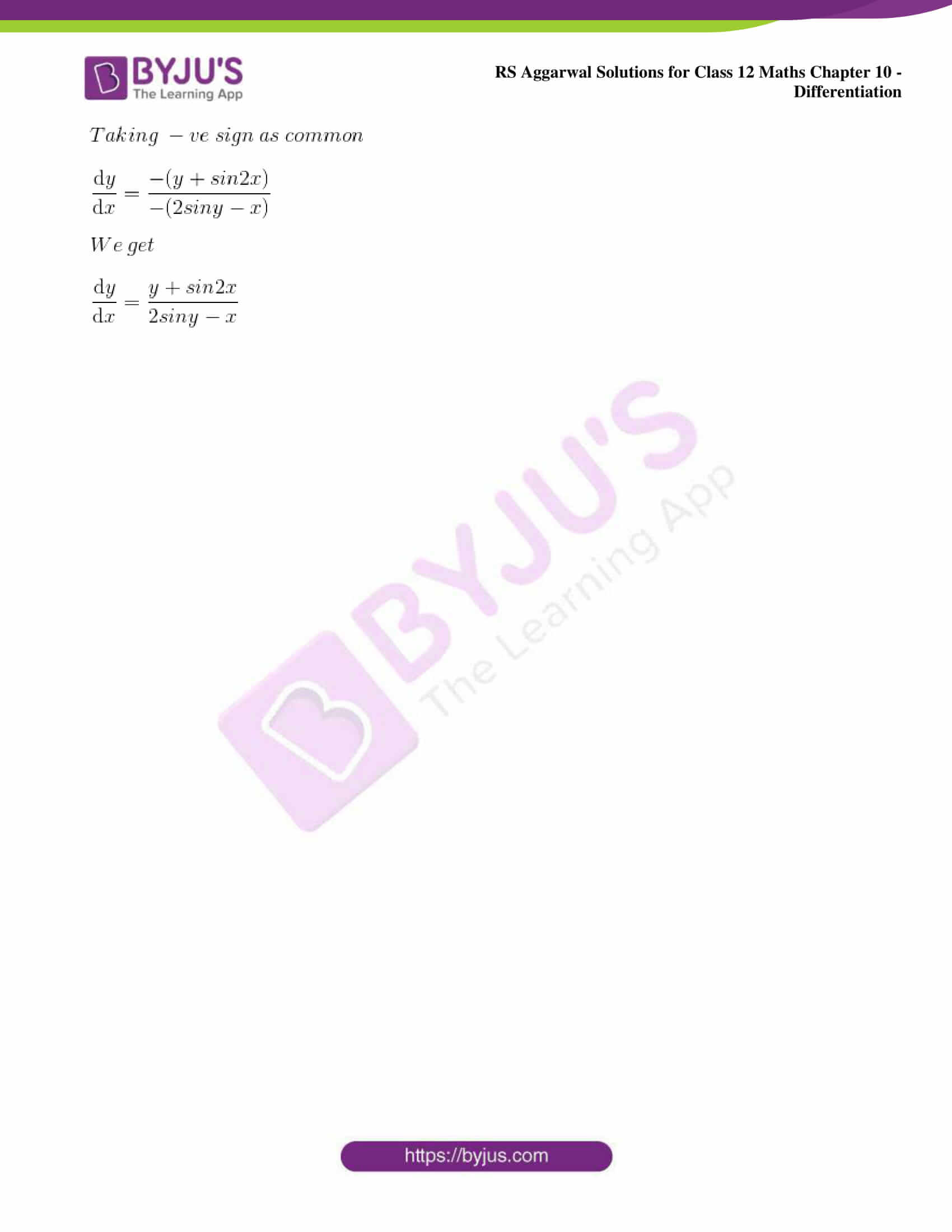### Access other exercise solutions of Class 12 Maths Chapter 10: Differentiation

Exercise 10A Solutions

Exercise 10B Solutions

Exercise 10C Solutions

Exercise 10D Solutions

Exercise 10F Solutions

Exercise 10G Solutions

Exercise 10H Solutions

Exercise 10I Solutions

Exercise 10J Solutions

### Access RS Aggarwal Solutions for Class 12 Maths Chapter 10: Differentiation Exercise 10E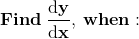1. x2 + y2 = 4

Solution: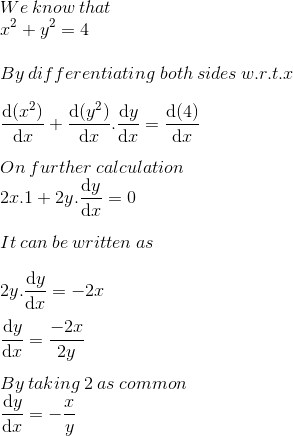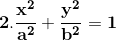Solution: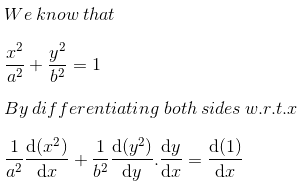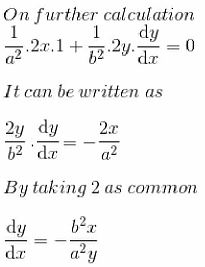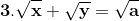Solution: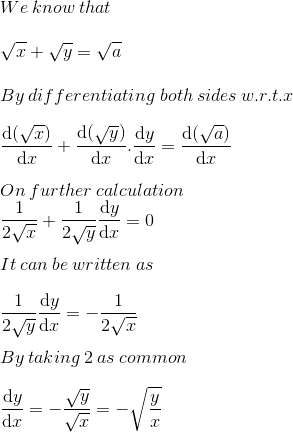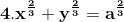Solution: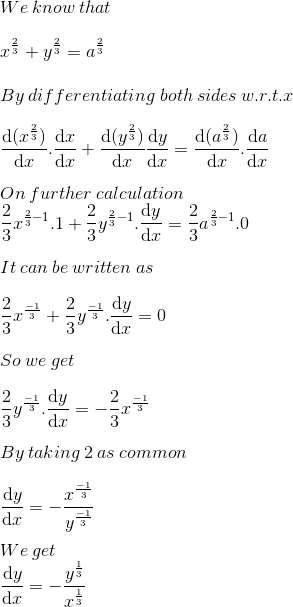5. xy = c2

Solution: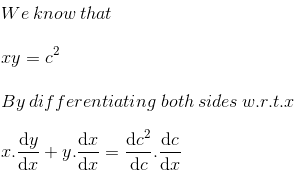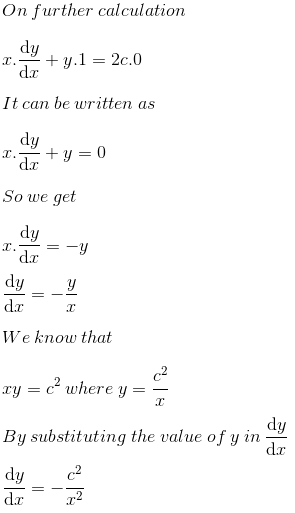6. x2 + y2 – 3xy = 1

Solution: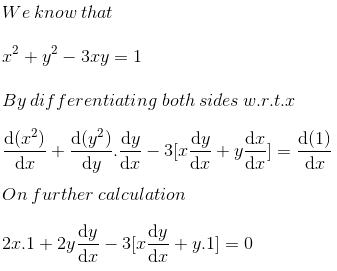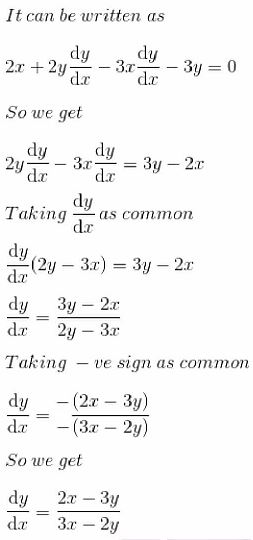7. xy2 – x2 y – 5 = 0

Solution: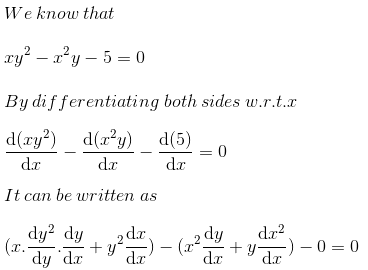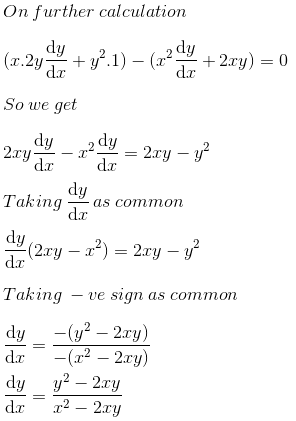8. (x2 + y2)2 = xy

Solution: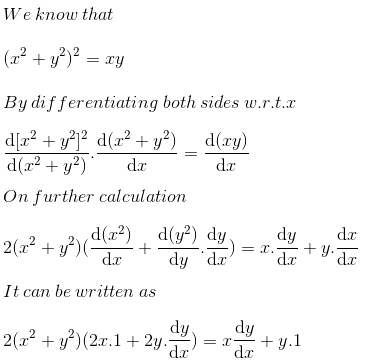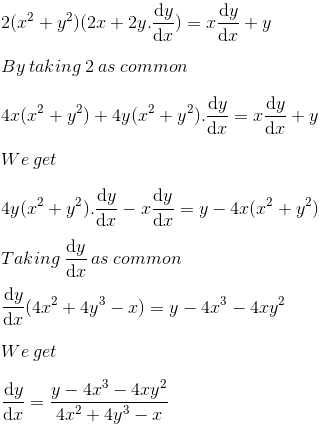9. x2 + y 2 = log (xy)

Solution: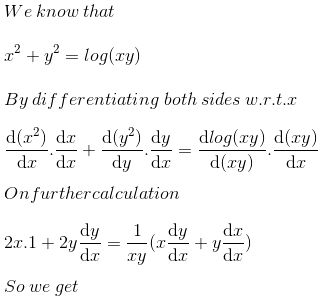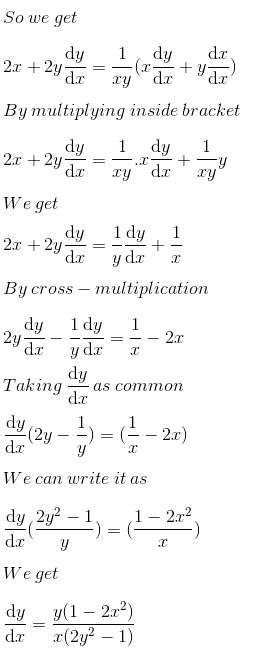10. xn + yn = an

Solution: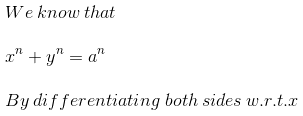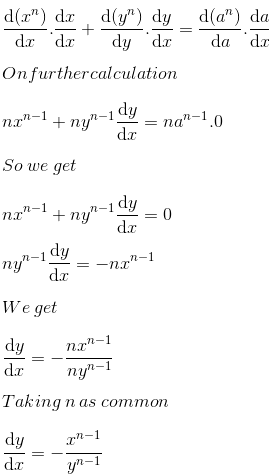11. x sin 2y = y cos 2x

Solution: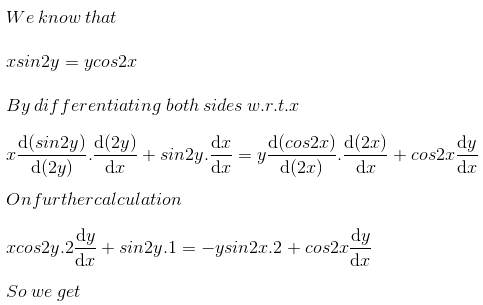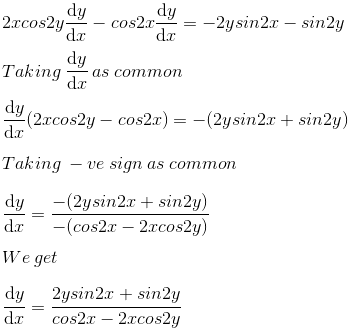12. sin2x + 2cos y + xy = 0

Solution: